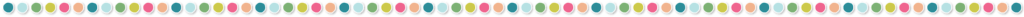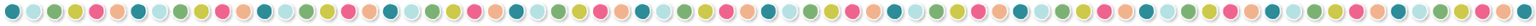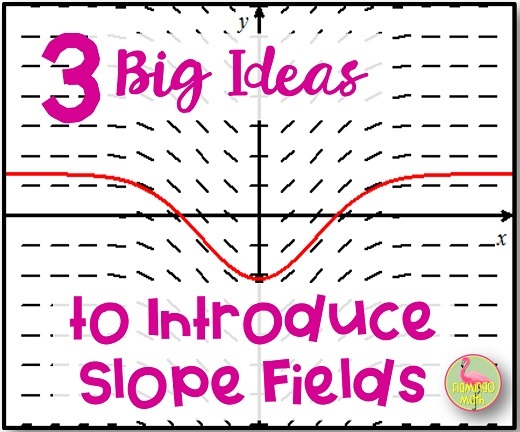# 3 Big Ideas to Introduce Slope FieldsDo you struggle to introduce SLOPE FIELDS to your students each year? The topic of slope fields was first introduced to the AP Calculus AB Course Description, in 2004. I recall confusion, in my own mind, about how I was going to introduce this concept back then.

First, we need to get a handle on the BIG PICTURE ! In teaching mathematics, we want students to incorporate multiple representations of all the major ideas, as they discover various methods to solve equations. Many call this the Rule of Four, or GNAW (Graphically, Numerically, Algebraically, and Verbally).

In AP Calculus AB, our main goal at this time of year, is to solve linear, first-order differential equations. To meet this goal, let’s incorporate lessons to help students make a connection to solving a “DE” algebraically with separation of variables, numerically with Euler’s Method, and graphically with slope fields.

Okay, so you ask, what is a Differential Equation?

A differential equation is an equation that gives you information about how the slope (dy/dx, or derivative) of some function family is related to the coordinates of points on the curve. In Calculus AB, we cover three types of Applications of Differential Equations for which students are responsible:

Type 1: Position, Velocity, Acceleration
Type 2: Growth and Decay, the simple case
Type 3: Growth and Decay where quantities change at a rate proportional to the difference between the existing amount of that quantity and some fixed constant B, such as Newton’s Law of Heating and Cooling.

Then, in Calculus BC, students will explore

Type 4: Logistic Differential Equations for Population modeling. Now that you have a handle on where we are headed, let’s go look at how and what creates a slope field.

What are slope fields? How can I introduce them to my class?

A slope field is the graphical representation of a differential equation in the GNAW Rule of Four approach. It is a graph of short line segments that are slopes tangent to the curve of the function at some point along the curve.

### BIG IDEA #1:

GRAPHICALLY:  Look at the family of curves for parabolas: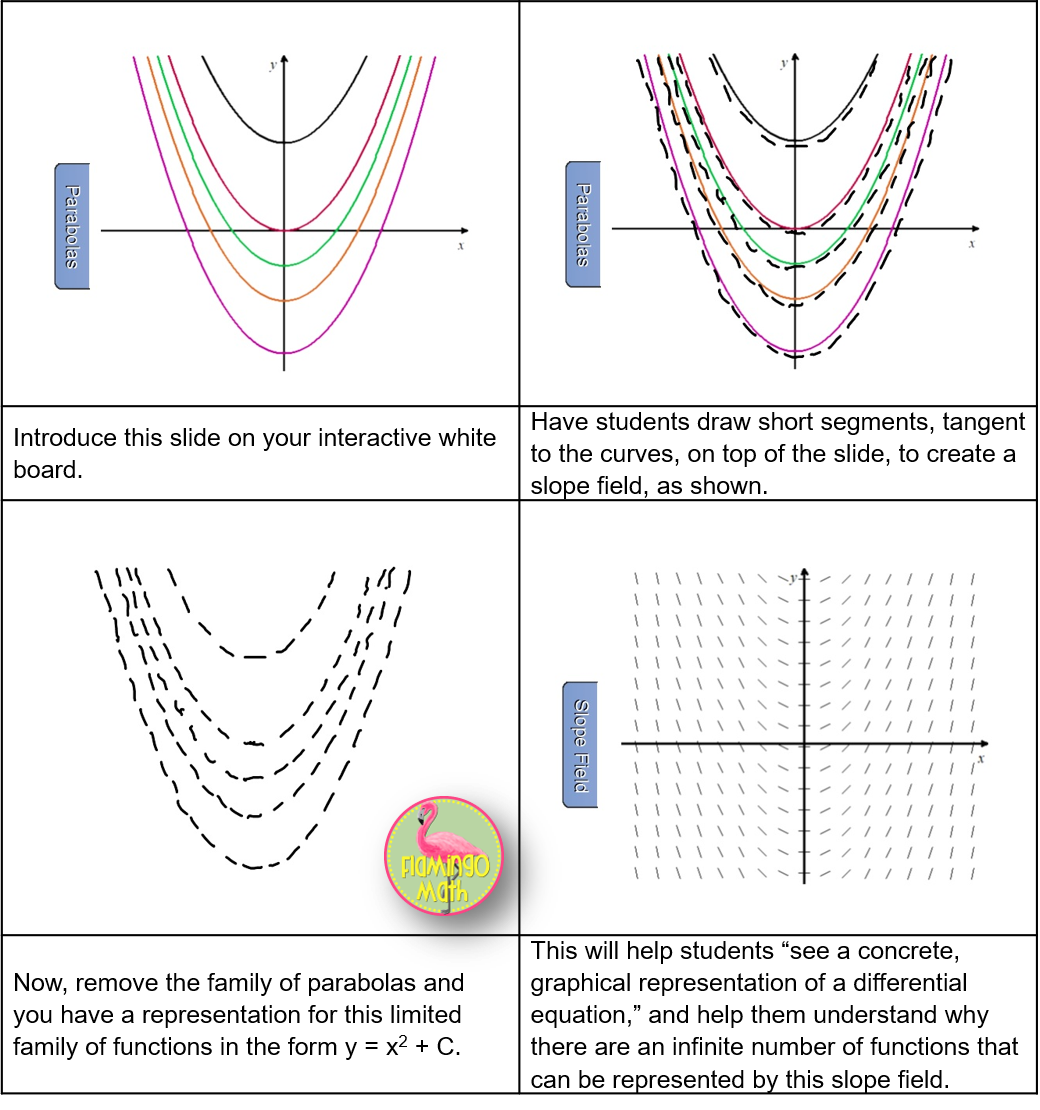### BIG IDEA #2:

NUMERICALLY:  Let’s consider the differential equation whose slope field is shown below.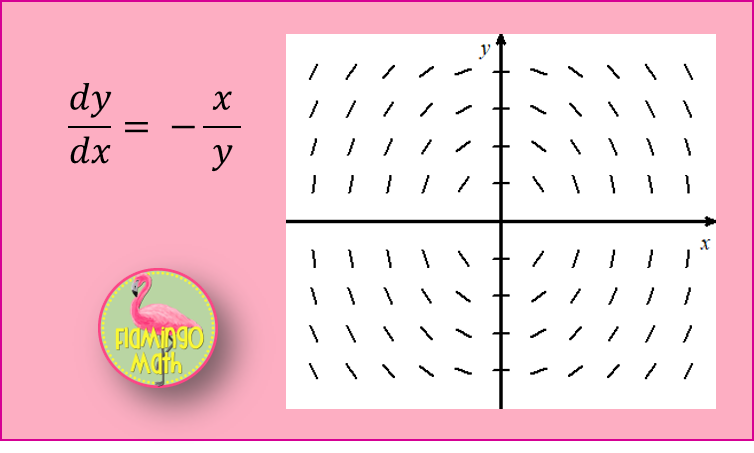Can you see the “image of a solution curve” hiding in the slopes shown? If you think of a slope field as a set of “signposts” pointing in the direction we should go at each “marker,” it becomes easy.

Give each student an ordered pair to evaluate the “slope” in the window from [-3, 3] by [-3, 3]. Have them sketch their slopes on a grid or use this slide to create a transparency for your document camera.

This is a rich opportunity to open the discussion about possible constraints that College Board might require during the AP Exam.

Some thoughtful questioning might include:

1. What solutions would constrain the slope to be horizontal? If y-values aren’t zero,
then x = 0 would be a possible solution.
2. How can the slopes approach vertical? As long as x-values aren’t zero, then as y approaches zero, the slopes are approaching vertical. Be sure to remind students that 0/0 isn’t 1, and, that solutions cannot pass through any undefined slopes, including vertical slopes. Will they know to restrict the domain at x = -3 and x = 3?
3. Are there any absolutes in this slope field? For example, the slope will ALWAYS BE NEGATIVE in Quadrants I and III, and ALWAYS POSITIVE in Quadrants II and IV.
4. How would you sketch the particular solution, y = f(x), that passes through the point (0, 3)? Will they recognize the fact that their answer must be a function? When reading the prompt that asks for y = f(x), do they know that their answer must pass the vertical line test, and will not include points where the slope (derivative) is undefined.

For our particular solution, with the initial condition of (0, 3), the solution is x2 + y2 = 9 for a domain of −3 < x < 3.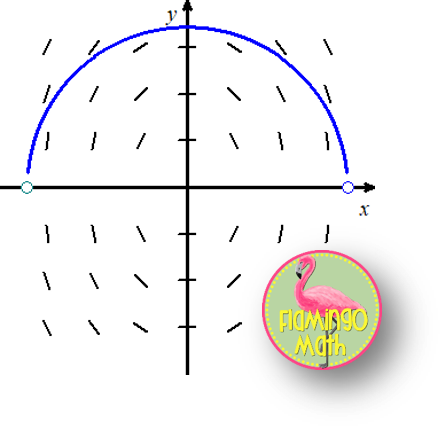### BIG IDEA #3:

TECHNIQUES FOR HOW TO DISTINGUISH BETWEEN SLOPE FIELDS:

While graphing calculators, DESMOS, GEOGEBRA,  and my favorite WINPLOT  will draw slope fields, students are not permitted to use technology on the AP Exam for this question stem. Students should know how to sketch slope fields by hand; and, know how to identify a slope field for given conditions or particular solutions.  How do you explain, guide, and direct your students to develop a technique for such situations?

What strategies and trends do you look for when matching slope fields?

• Look for places where the slopes are 0; that is, dy/dx=0
• Look for places where the slopes are undefined
• Look at the slopes along the y-axis
• Look to see if the slopes only depend on x; vertical translations
• Look to see if the slopes only depend on y; horizontal translations
• Is the derivative changing on intervals of x, y, or both? If it’s only changing on one variable then the rate (dy/dx) will only contain that variable.
• Look to see where the slopes are positive and where they are negative
• As a last resort, plug in random points. Such as, (1, 1) (1, -1) (-1, -1) and (-1, 1) for example. You want to eliminate possible answers.

Finally, I’ve created a special Slope Fields Matching Practice Activity for you here. Be sure to check out the HOT TOPICS: Introducing Slope Fields Lesson that follows this blog post.

Never miss a blog post, be sure to subscribe to the Newsletter for more engaging posts, and a FREE Infographic for SLOPE FIELDS.

### Subscribe to our mailing list!

We will send you the FREE SLOPE FIELD INFOGRAPHIC